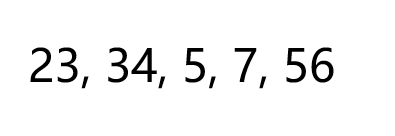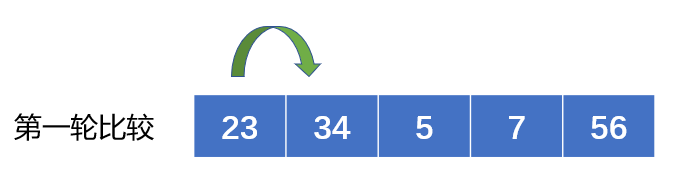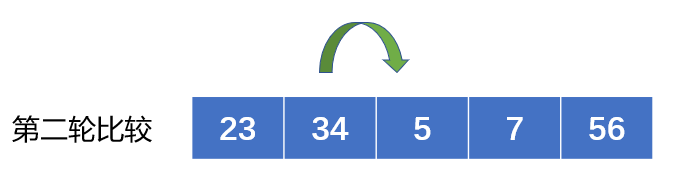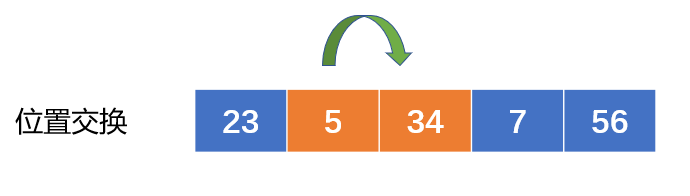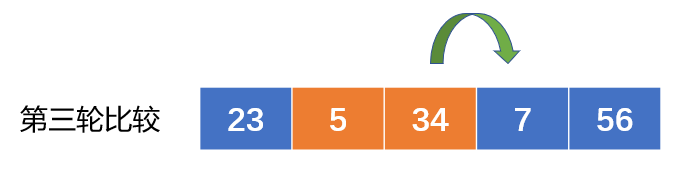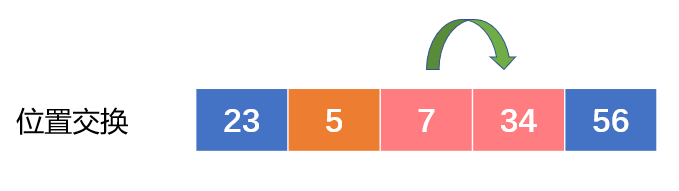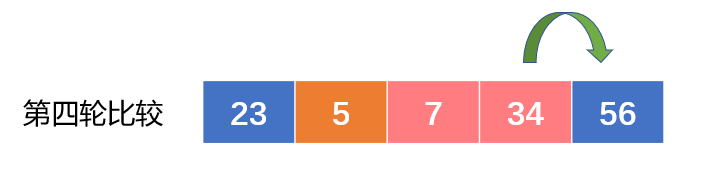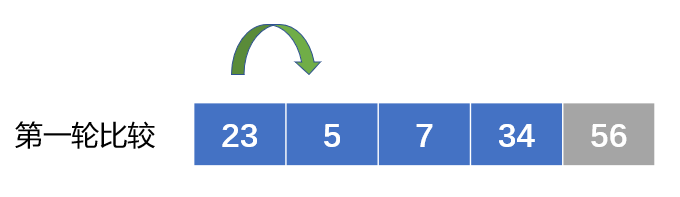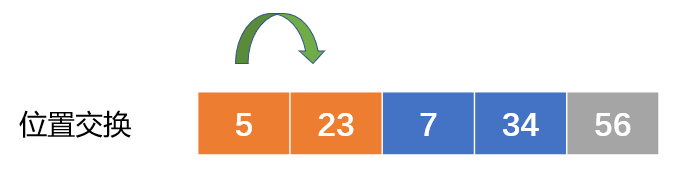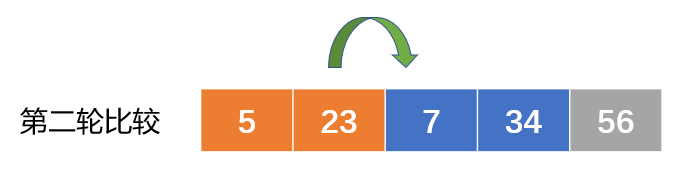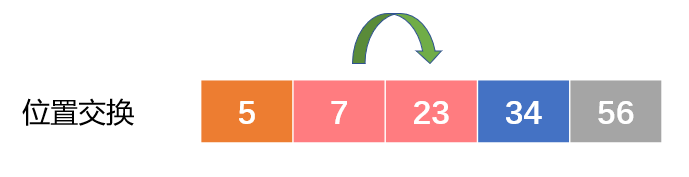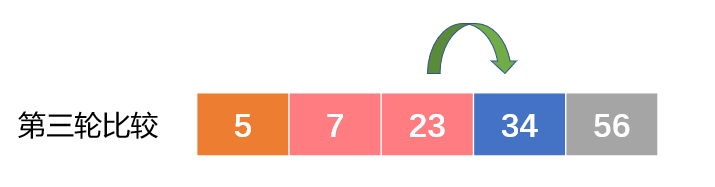python实现冒泡排序

```def bubbleSort(arr):
n = len(arr)
# 遍历所有数组元素
for i in range(n):
for j in range(0, n-i-1):
if arr[j] > arr[j+1]:
arr[j], arr[j+1] = arr[j+1], arr[j]

arr = [64, 34, 25, 12, 22, 11, 90]

bubbleSort(arr)

print ("排序后的数组:")
for i in range(len(arr)):
print ("%d" %arr[i])``````public class SortSample {

/**
* @Title: main
* @Description:Java程序入口main方法
* @param @param args 参数
*
* @return void 返回类型
* @throws
*/

public static void main(String[] args) {
/**
* 声明长度为10的一维数组，
* 存储待排序的数值 数组类型是int
*/
int arr[] = new int;
int i;
Scanner sc = new Scanner(System.in);
System.out.printf("请输入 %d 个整数", arr.length);
// 使用for循环，接收用户输入的整数
for (i = 0; i < arr.length; i++) {
System.out.printf("\n 请输入第 %d 个整数：", i + 1);
arr[i] = sc.nextInt();

}
System.out.print("\n ***开始排序***");
/**
* 控制排序趟数
* 有多少个待排序的数值就扫描数组多少趟
*  针对每个待排序数值都要和其它数值进行比较
*/
for (i = 0; i < arr.length - 1; i++) {
/**
* 控制每一趟排序多少次
* 每一趟排序都会把较大的数值移动到数组后面
* 第i趟排序循环次数为数组长度减去已经排过序的i个元素
* 如果第j个元素大于第j+1个元素，则交换
*/
for (int j = 0; j < arr.length - 1 - i; j++) {
if (arr[j] > arr[j + 1]) {
int temp = arr[j];
arr[j] = arr[j + 1];
arr[j + 1] = temp;
}
}
}
System.out.print("\n ***排序完成***\n");
System.out.println("排序后的数组为：");
for (i = 0; i < arr.length; i++) {
System.out.print(arr[i] + ",");
}

}

}```

• 备案号：鲁ICP备15001146号
• @1997-2018 潍坊米粒花网络技术有限公司版权所有